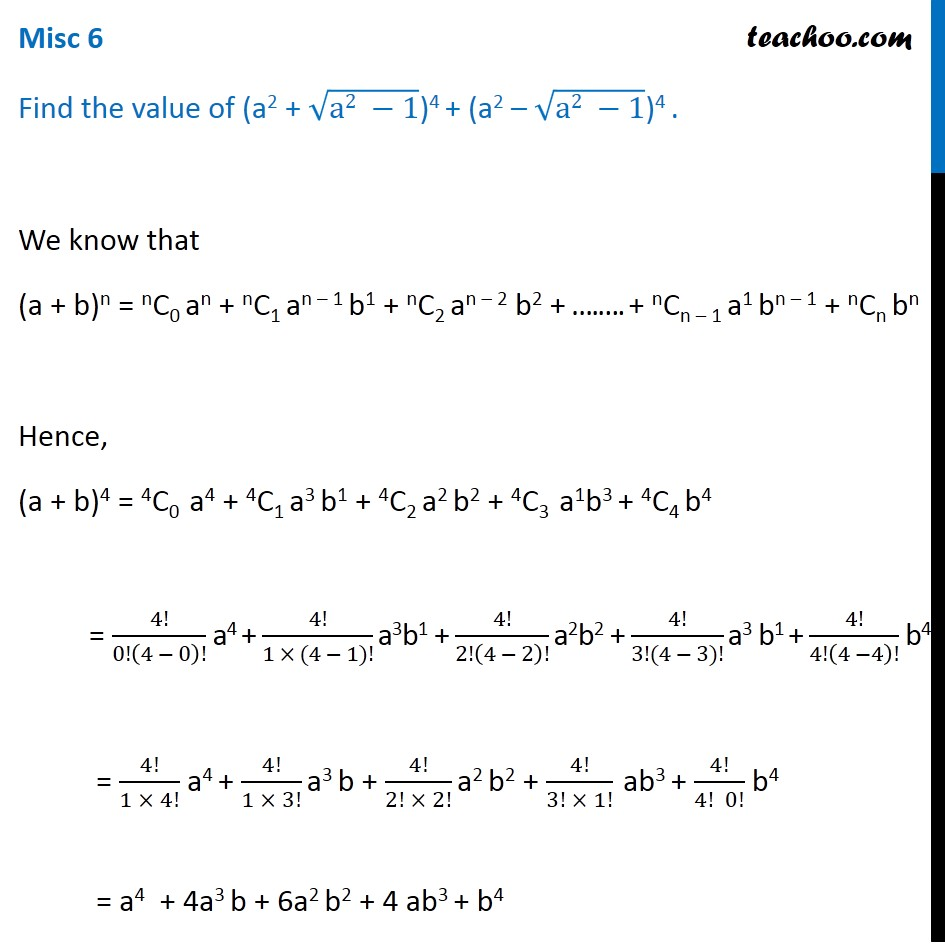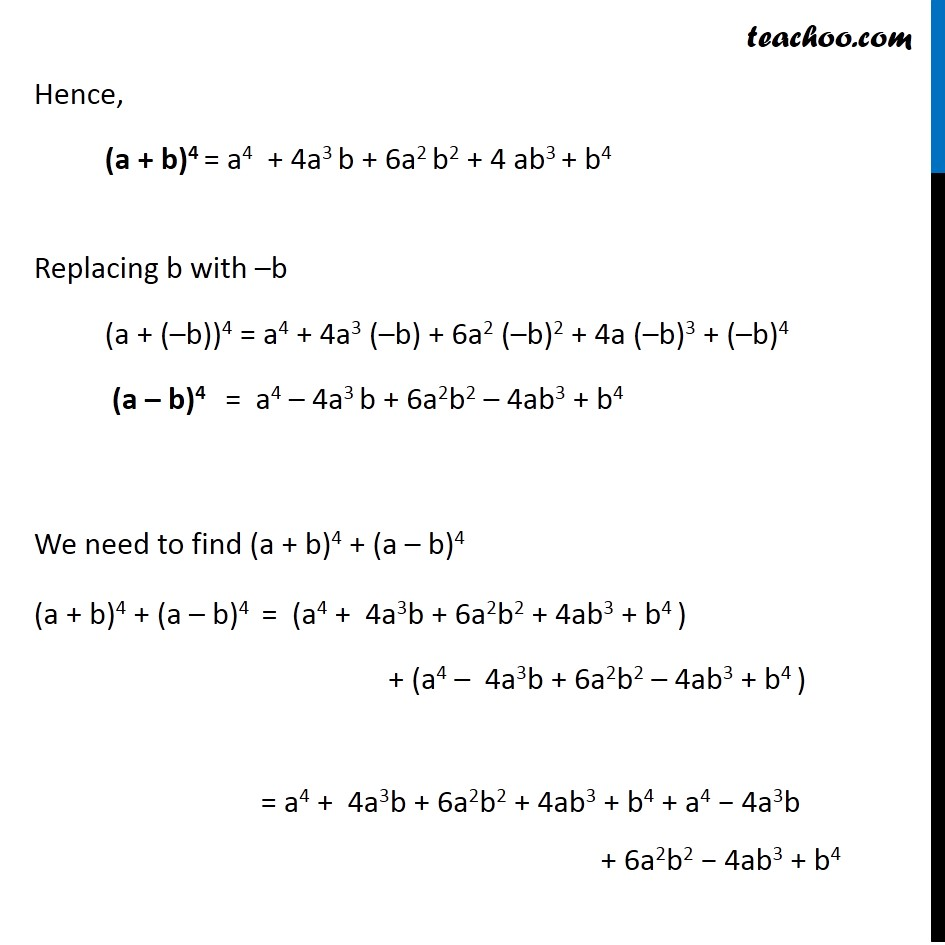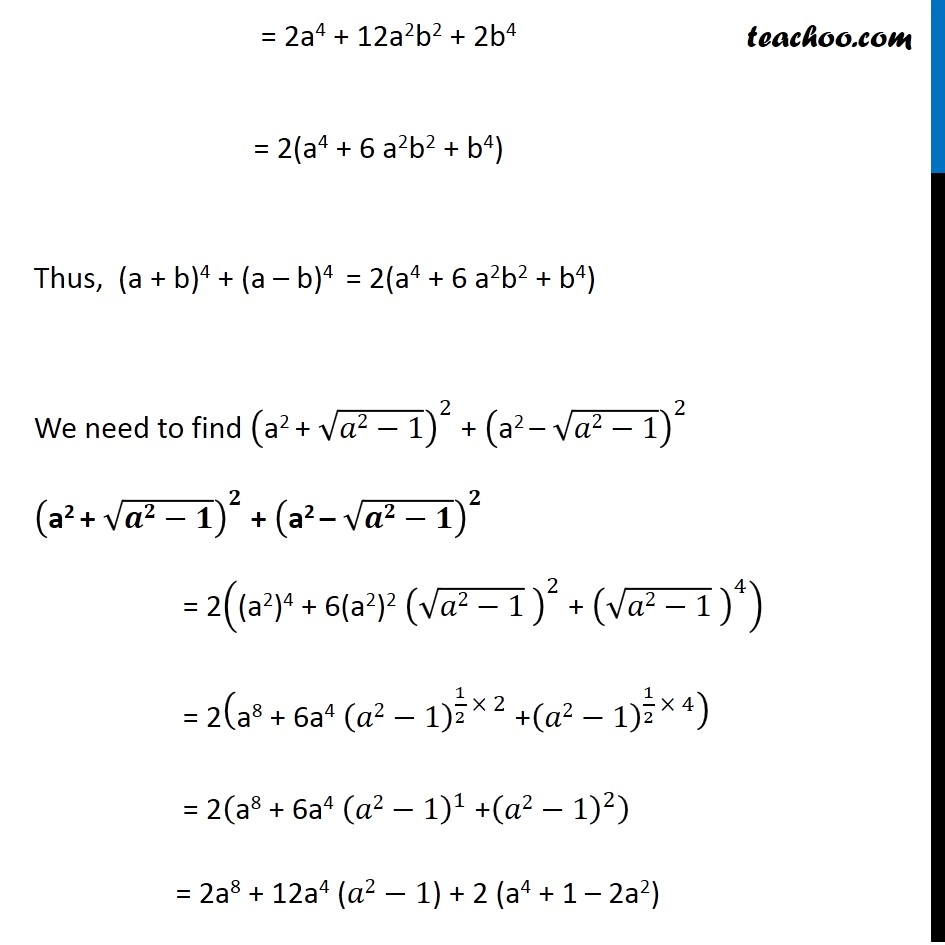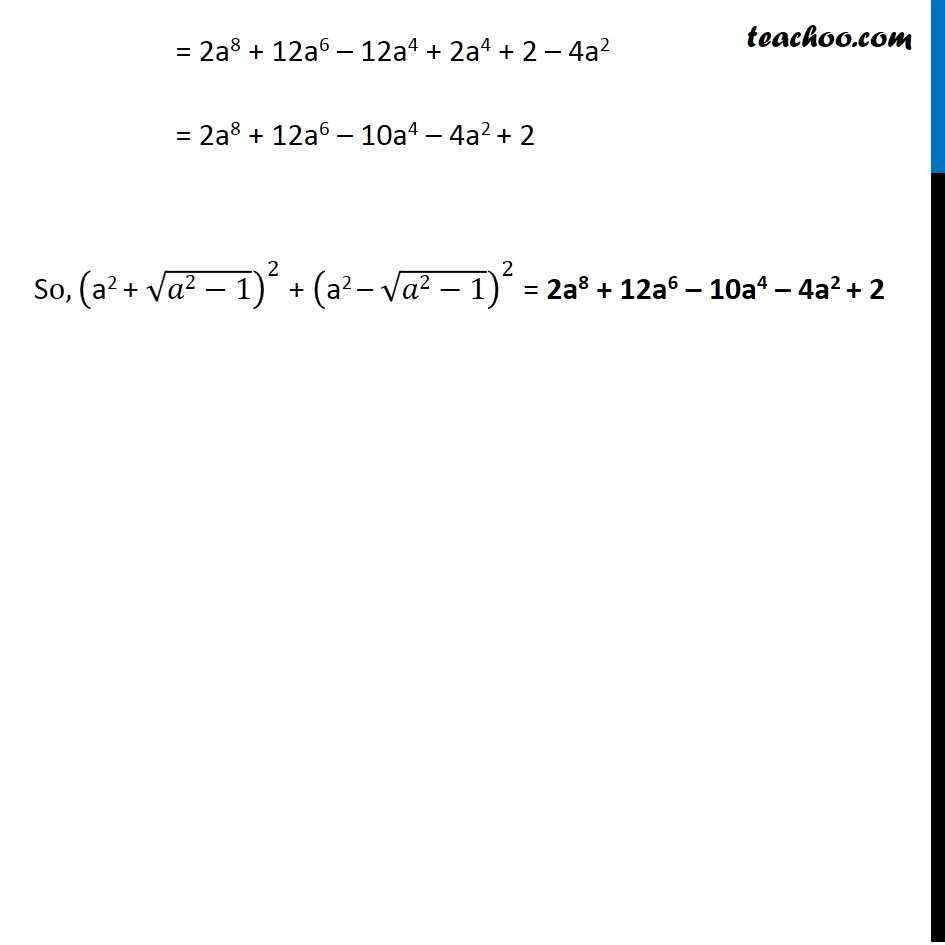1. Chapter 8 Class 11 Binomial Theorem (Deleted)
2. Concept wise
3. Expansion

Transcript

Misc 6 Find the value of (a2 + √(a2 −1))4 + (a2 – √(a2 −1))4 . We know that (a + b)n = nC0 an + nC1 an – 1 b1 + nC2 an – 2 b2 + ….…. + nCn – 1 a1 bn – 1 + nCn bn Hence, (a + b)4 = 4C0 a4 + 4C1 a3 b1 + 4C2 a2 b2 + 4C3 a1b3 + 4C4 b4 = 4!/0!(4 − 0)! a4 + 4!/(1 × (4 − 1)!) a3b1 + 4!/2!(4 − 2)! a2b2 + 4!/(3!(4 − 3)!) a3 b1 + 4!/4!(4 −4)! b4 = 4!/(1 × 4!) a4 + 4!/(1 × 3!) a3 b + 4!/(2! × 2!) a2 b2 + 4!/(3! × 1!) ab3 + 4!/(4! 0!) b4 = a4 + 4a3 b + 6a2 b2 + 4 ab3 + b4 Hence, (a + b)4 = a4 + 4a3 b + 6a2 b2 + 4 ab3 + b4 Replacing b with –b (a + (–b))4 = a4 + 4a3 (–b) + 6a2 (–b)2 + 4a (–b)3 + (–b)4 (a – b)4 = a4 – 4a3 b + 6a2b2 – 4ab3 + b4 We need to find (a + b)4 + (a – b)4 (a + b)4 + (a – b)4 = (a4 + 4a3b + 6a2b2 + 4ab3 + b4 ) + (a4 – 4a3b + 6a2b2 – 4ab3 + b4 ) = a4 + 4a3b + 6a2b2 + 4ab3 + b4 + a4 − 4a3b + 6a2b2 − 4ab3 + b4 = 2a4 + 12a2b2 + 2b4 = 2(a4 + 6 a2b2 + b4) Thus, (a + b)4 + (a – b)4 = 2(a4 + 6 a2b2 + b4) We need to find ("a2 + " √(𝑎2−1))^2 + ("a2 – " √(𝑎2−1))^2 ("a2 + " √(𝒂𝟐−𝟏))^𝟐 + ("a2 – " √(𝒂𝟐−𝟏))^𝟐 = 2("(a2)4 + 6(a2)2 " (√(𝑎2−1) )^2 " + " (√(𝑎2−1) )^4 ) = 2("a8 + 6a4 " (𝑎2−1)^(1/2 × 2) " +" (𝑎2−1)^(1/2 × 4) ) = 2("a8 + 6a4 " (𝑎2−1)^1 " +" (𝑎2−1)^2 ) = 2a8 + 12a4 (𝑎2−1) + 2 (a4 + 1 – 2a2)a = 2a8 + 12a6 – 12a4 + 2a4 + 2 – 4a2 = 2a8 + 12a6 – 10a4 – 4a2 + 2 So,("a2 + " √(𝑎2−1))^2 + ("a2 – " √(𝑎2−1))^2 = 2a8 + 12a6 – 10a4 – 4a2 + 2

Expansion

Chapter 8 Class 11 Binomial Theorem (Deleted)
Concept wise